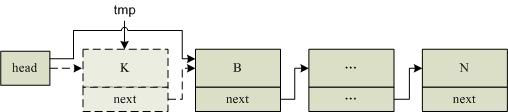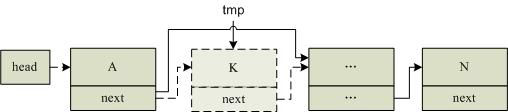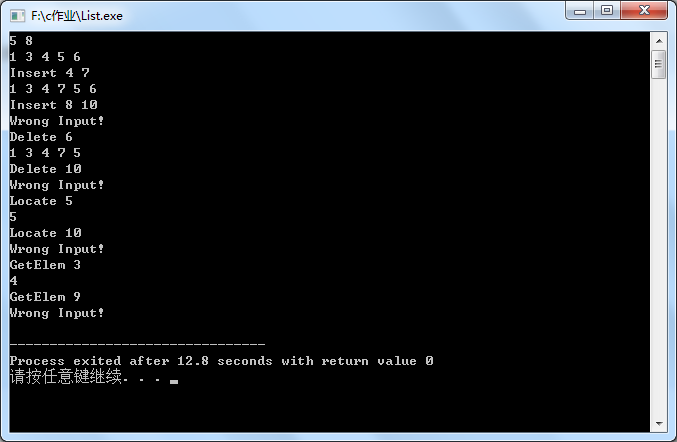• 错误示范 struct node * DelNode(struct node*head,int x) {  if (head==NULL) //1、空链表  {  printf("空链表，无可删\n");  exit(0);  }  struct node* p=head;
错误示范

struct node * DelNode(struct node*head,int x)
{
if (head==NULL)                     //1、空链表
{
printf("空链表，无可删\n");
exit(0);
}
struct node* p=head;
struct node* pre=NULL;
int find=0;
while (p!=NULL)
{
if (p->data==x)
{
find = 1;
break;
}
pre = p;                        //pre记录目标节点的前一个
p=p->next;
}
if (find==0)                        //2、没有要找的节点
{
printf("No this data\n");
exit(0);
}
if (p->next==NULL)                        //要找的节点在末尾
{
if (pre==NULL)  //只有一个节点
{
head = NULL;
}
else
{
pre->next = NULL;//末尾
}
free(p);
}
else    //要找的不在末尾  这种处理  能包含首节点吗？？当然不能   首节点则pre==NULL
{
pre->next=p->next;
free(p);
}
return head;
}

分几类
/*
1、空链表
2、没有找到目标
3、首节点
4、尾节点
5、中间节点
注意 首节点的处理是让head=head->next;包含了只有一个节点的情况
*/


展开全文• 这是一个删除结点并输出的程序，总共有三个链表 错误为：当删除最后一个链表也就是3时程序发生错误，停止运行。 求大家帮忙解解决!  #include <stdio.h> #define NUll 0 #include struct stu { char name;...c
• 删除节点就是将某一节点从链中摘除。 将待删节点与其前一节点解除联系（中间或尾部）或本阶段删除（头节点），并释放相应空间(free)。删除的第一步是找到要删除的节点，同链表查找，如果找不到或链表为空，提示未...
链表的创建查看
删除节点就是将某一节点从链中摘除。
将待删节点与其前一节点解除联系（中间或尾部）或本阶段删除（头节点），并释放相应空间(free)。删除的第一步是找到要删除的节点，同链表查找，如果找不到或链表为空，提示未找到，找到后根据情况删除此节点。删除节点两种情况：第一个节点，后面节点。
步骤：
1、链表为空：不用删除
2、链表不为空：先循环找要删除的节点1）找到了

1>找到的节点是头节点
被删除节点是第一个节点：只需使head指向第二个节点即可2>找到的节点是普通节点
被删节点不是第一个节点：使被删节点的前一节点指向被删节点的后一节点即可2）没找到
结构体节点：
typedef struct student {
int num;        //学号
int score;    //分数
char name;
struct student *next;//指针域
}STU;demo：
1、
void link_delete_num(STU **p_head,int num)
{
STU * pb,*pf;
pb=*p_head;//让pb指向头节点
if(*p_head == NULL)//链表为空链表
{
printf("链表为空\n");
return ;
}
while(pb->num !=num && pb->next!=NULL)//循环查找要删除的节点
{
pf=pb;
pb=pb->next;
}
if(pb->num == num)//找到了一个节点的num和num相等
{
if(pb == *p_head)//找到的节点是头节点
{
*p_head = pb->next;
}
else//找到的节点是普通节点
{
pf->next = pb->next;
}
free(pb);
}
else//没有找到咱们要删除的节点
{
printf("没有找到您要删除的节点\n");
}
}

2、
void link_delete_name(STU **p_head,char *name)
{
STU * pb,*pf;
pb=*p_head;//让pb指向头节点
if(*p_head == NULL)//链表为空链表
{
printf("链表为空\n");
return ;
}
while(strcmp(pb->name,name)!=0 && pb->next!=NULL)//循环查找要删除的节点
{
pf=pb;
pb=pb->next;
}
if(strcmp(pb->name,name)==0)//找到了一个节点的num和num相等
{
if(pb == *p_head)//找到的节点是头节点
{
*p_head = pb->next;
}
else//找到的节点是普通节点
{
pf->next = pb->next;
}
free(pb);
}
else//没有找到咱们要删除的节点
{
printf("没有找到您要删除的节点\n");
}
}

展开全文• C语言通过链表指针删除链表节点的算法C语言通过链表指针删除链表节点的算法完整源码(定义，实现，main函数测试） C语言通过链表指针删除链表节点的算法完整源码(定义，实现，main函数测试） #include <iostream&...
C语言通过链表指针删除链表节点的算法C语言通过链表指针删除链表节点的算法完整源码(定义，实现，main函数测试）
C语言通过链表指针删除链表节点的算法完整源码(定义，实现，main函数测试）
#include <iostream>

struct Node {
int data;
Node * next;
Node( int d ) : data{ d }, next{ nullptr } { }
};

void insert( Node * & head, int data )
{
Node *newNode = new Node(data);
if ( head == nullptr ) {
head = newNode;
} else {
Node * temp = head;
while ( temp->next != nullptr ) {
temp = temp->next;
}
temp->next = newNode;
}
}

void printList( Node * head )
{
while( head ) {
std::cout << head->data << "-->";
head = head->next;
}
std::cout << "NULL" << std::endl;
}

void deleteNode( Node * node )
{
// return if node is null
if ( node == nullptr ) {
return;
}

//this method won't work if we are given last node
if ( node->next == nullptr ) {
return;
}

Node * nextNode = node->next;
node->data = nextNode->data;
node->next = nextNode->next;
delete nextNode;
}

int main()
{
Node * head = nullptr;
insert( head, 1 );
insert( head, 12 );
insert( head, 2 );
insert( head, 3 );
insert( head, 4 );
printList( head );
std::cout << "Deleting node with data 12 \n";
deleteNode( head->next );
printList( head );
return 0;
}



展开全文• #include <stdio.h> #define NUll 0 #include struct stu { char name; int xuehao; int grade;... struct stu *next;...错误为：当删除最后一个链表也就是3时程序发生错误，停止运行。 求解c
• 数据结构c语言链表删除重复节点，包含数据类型、结构的定义和函数的实现
• Delete X：删除顺序表中的X元素，如果有多个X元素，只删除第一个X，遍历输出当前顺序的所有元素。 Locate X：输出顺序表中X元素的所在的位置，如果有多个X元素，只输出第一个X元素的位置。 GetElem X：输出顺序表中X...
线性表操作
顺序表是我们数据结构中的基本储存形式，现在给定一个顺序表，有如下操作：
Insert X Y：在顺序表中X位置插入Y元素，遍历输出当前顺序表的所有元素。
Delete X：删除顺序表中的X元素，如果有多个X元素，只删除第一个X，遍历输出当前顺序的所有元素。
Locate X：输出顺序表中X元素的所在的位置，如果有多个X元素，只输出第一个X元素的位置。
GetElem X：输出顺序表中X位置上的元素。
代码如下：
#include<string.h>
#include<stdio.h>
#include<stdlib.h>
typedef struct node                         //定义一个结构体
{
int data;
struct node *next;
}node, *lk;

void init(lk *l)                                 //定义一个链表头节点
{
(*l) = (lk)malloc(sizeof(node));
(*l)->next = NULL;
}
void creat(lk l,int n)                          //创建一个链表
{
int a;
lk tmp, p;
tmp = l;
int i;
for(i = 0;i < n;i++)
{
p = (lk)malloc(sizeof(node));
scanf("%d", &a);
p->data = a;
p->next = NULL;
tmp->next = p;
tmp = p;
}
}
void insert(lk l,int n, int m)            //Insert X Y：在顺序表中X位置插入Y元素，遍历输出当前顺序表的所有元素。
{
lk p, q;
p = l;
int i;
for(i = 0;i < n-1;i++)
{
p = p->next;
}
q = (lk)malloc(sizeof(node));
q->data = m;
q->next = p->next;
p->next = q;
}
void show(lk l)    //打印链表
{
int flag = 1;
lk p;
p = l;
p = p->next;
while(p)
{
if(flag)
{
printf("%d", p->data);
flag = 0;
}
else{
printf(" %d", p->data);
}
p = p->next;
}
printf("\n");
}
int GetElem(lk l, int n)     //GetElem X：输出顺序表中X位置上的元素。
{
int i;
for(i = 0;i < n;i++)
{
l = l->next;
}
return l->data;
}
int Delete (lk l, int n)               //Delete X：删除顺序表中的X元素，如果有多个X元素，只删除第一个X，遍历输出当前顺序的所有元素。
{
lk p ,q,s;
p = l;
q = l->next;
int i = 0;
while(q)
{
if(q->data==n)
{
p->next = q->next;
//s = q;
q= q->next;
i = 1;
break;
}
else
{
p = p->next;
q = q->next;
}
}
return i;
}
int Locate(lk l,int n)   Locate X：输出顺序表中X元素的所在的位置，如果有多个X元素，只输出第一个X元素的位置。
{
int q = 0;
while(1)
{
l = l->next;
q++;
if(l->data == n)
{
break;
}
}
return q;
}
int main()
{
int m , n;
scanf("%d %d", &m, &n);
lk l;
init(&l);
creat(l, m);
int k;
char a;
int x,y,rt;
for(k = 0;k < n;k++)
{
scanf("%s", &a);
if(a == 'I')
{
scanf("%d %d", &x, &y);
if(x > m)
{
printf("Wrong Input!\n");
}
else
{
insert(l,x,y);
show(l);
}
}
else if(a == 'D')
{
scanf("%d", &x);
if(Delete(l,x) == 0)
{
printf("Wrong Input!\n");
}
else
{
Delete(l,x);
show(l);
}
}
else if(a == 'L')
{
scanf("%d", &x);
if(x > m)
{
printf("Wrong Input!\n");
}
else
{
rt = Locate(l,x);
printf("%d\n", rt);
}
}
else if(a == 'G')
{
scanf("%d", &x);
if(x > m)
{
printf("Wrong Input!\n");
}
else
{
rt = GetElem(l,x);
printf("%d\n", rt);
}
}
else
{
printf("%d\n", rt);
}
}
return 0;
}

结果展示：`
展开全文插入
• #include<stdio.h> #include<stdlib.h> #include<string.h>...函数功能：新建节点 返回值：struct * 参数：int data */ struct mylist* create_node(int data); /* 函数名：insert_hea.
• 静态链表节点删除 #include <stdio.h> //#include<stdiob.h> struct student{ int yu; int shu; int ying; struct student *next; }; void printlink(struct student *head){ //先while遍历时...算法
• 头指针链表与头节点链表 二者差异 头指针是指指向开始结点的指针...对于头指针链表，有了头结点后，对在第一个元素结点前插入结点和删除第一个结点，其操作与对其它结点的操作统一了。 以下分别为对头指针...
• C语言静态链表（指定节点的前后插入新节点删除
• 链表可以动态地进行存储分配，因此可将其看做一个功能强大的数组，在节点中可以定义多种数据类型，还可以根据需要修改、增加、删除节点链表都有一个头指针，一般以 head 来表示，存放的是一个地址。链表中的节点...
• C语言实现删除链表所有节点空间 节点结构 struct node { int data; node *next; }; 删除函数 //释放链表节点空间 void delete_chain(node *head) { node *p1; while (head) { p1 = head; head = head->...
• 删除链表中的节点(C语言)(C语言) 题目： 请编写一个函数，使其可以删除某个链表中给定的（非末尾）节点，你将只被给定要求被删除节点。 现有一个链表 – head = [4,5,1,9]，它可以表示为: 示例1： 输入: ...LeetCode
• 请编写一个函数，使其可以删除某个链表中给定的（非末尾）节点，你将只被给定要求被删除节点。 现有一个链表 --head =[4,5,1,9]，它可以表示为: 示例 1: 输入: head = [4,5,1,9], node = 5 输出: [4,1,9] ...
• 删除链表中的节点 请编写一个函数，使其可以删除某个链表中给定的（非末尾）节点。传入函数的唯一参数为 要被删除节点 。 现有一个链表 – head = [4,5,1,9]，它可以表示为: 示例 1： 输入：head = [4,5,1,9], ...
• 链表节点 struct node{ int data; struct node* nxt; }; typedef struct node Node; 顺序创建链表 Node* createLinkList(int n){ Node* head = (Node*) malloc(sizeof(Node)); Node* end =
• 请编写一个函数，使其可以删除某个链表中给定的（非末尾）节点。传入函数的唯一参数为 要被删除节点 。 现有一个链表 – head = [4,5,1,9]，它可以表示为: 示例 1： 输入：head = [4,5,1,9], node = 5 输出：[4,1...
• 关于这个问题，基本思路是先将要被删除节点的上一个节点和下一个节点连接起来，然后再把要删除的节点释放掉就行了。 首先先定义一个链表： struct STU { int a; int b; int c; struct STU *next; } struct STU ...
• 请编写一个函数，使其可以删除某个链表中给定的（非末尾）节点，你将只被给定要求被删除节点。 现有一个链表 – head = [4,5,1,9]，它可以表示为: 4 -&gt; 5 -&gt; 1 -&gt; 9 示例 1: 输入: head = [4...
• /*建立单链表 */ #include "stdio.h" #include "stdlib.h"  typedef struct node {  int data;  struct node * p_next;  }Node; ... Pnode creatnode(Pnode phead) //建立节点 { P
• 请编写一个函数，使其可以删除某个链表中给定的（非末尾）节点。传入函数的唯一参数为 要被删除节点 。 现有一个链表 -- head = [4,5,1,9]，它可以表示为: 示例 1： 输入：head = [4,5,1,9], node = 5 ...
• 目录 一、单向链表数据结构 二、单向链表增删改查函数声明 ...五、节点删除 六、链表清空 七、链表销毁 八、验证程序 一、单向链表数据结构 struct singal_list_node { struct singal_list_node...
• 思路：这个题刚刚看以为就是普通链表删除，但是发现题目没有给头节点，看了其他的解答发现思路大致是将node的后一个节点赋值给node,跳过node的一下个节点，因为要删除节点已经给出，所以不需要手动删除。...数据结构 leetcode
• 请编写一个函数，使其可以删除某个链表中给定的（非末尾）节点，你将只被给定要求被删除节点。 现有一个链表 --head =[4,5,1,9]，它可以表示为: 4 -> 5 -> 1 -> 9 示例 1: 输入: head = [4,5,1,9...
• 目录 二、双向链表增删改查函数声明 ...五、节点删除 六、链表清空 七、链表销毁 八、验证程序 一、双向链表数据结构 struct double_list_node { struct double_list_node *prev; /* pr...
• C语言实现删除链表的一个节点 节点表示 struct node { int data; node *next; }; 删除函数 //删除链表的一个节点 node *delete_node(node *head, int data) { node *p1, *p2; if (head == NULL) {//链表为空...
• 数据结构——单链表、双链表c语言实现 链表 特点（缺点）：（1）非连续结构，实现结构复杂（2）不支持随机访问 使用场景：频繁插入删除 链表类型：单向、双向；带头、不带头；循环、非循环 三种属性可组成8中...数据结构
• 单链表的实现细节3.1节点的创立3.2先来一个静态链表3.3动态链表的创建与插入3.4动态链表删除3.5链表为什么要有个表头（header）4.常见的问题5.双链表6.循环链表7.有关期末考试8.放在最后9.参考资料 0.创作背景与...数据结构...

# 链表c语言删除节点c语言 订阅